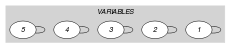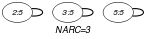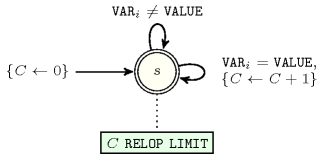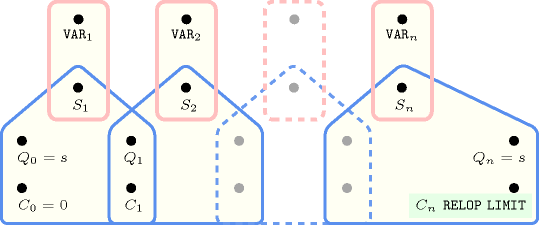## 5.91. count

Origin
Constraint

$\mathrm{𝚌𝚘𝚞𝚗𝚝}\left(\mathrm{𝚅𝙰𝙻𝚄𝙴},\mathrm{𝚅𝙰𝚁𝙸𝙰𝙱𝙻𝙴𝚂},\mathrm{𝚁𝙴𝙻𝙾𝙿},\mathrm{𝙻𝙸𝙼𝙸𝚃}\right)$

Synonyms

$\mathrm{𝚘𝚌𝚌𝚞𝚛𝚎𝚗𝚌𝚎𝚖𝚊𝚡}$, $\mathrm{𝚘𝚌𝚌𝚞𝚛𝚎𝚗𝚌𝚎𝚖𝚒𝚗}$, $\mathrm{𝚘𝚌𝚌𝚞𝚛𝚛𝚎𝚗𝚌𝚎}$.

Arguments
 $\mathrm{𝚅𝙰𝙻𝚄𝙴}$ $\mathrm{𝚒𝚗𝚝}$ $\mathrm{𝚅𝙰𝚁𝙸𝙰𝙱𝙻𝙴𝚂}$ $\mathrm{𝚌𝚘𝚕𝚕𝚎𝚌𝚝𝚒𝚘𝚗}\left(\mathrm{𝚟𝚊𝚛}-\mathrm{𝚍𝚟𝚊𝚛}\right)$ $\mathrm{𝚁𝙴𝙻𝙾𝙿}$ $\mathrm{𝚊𝚝𝚘𝚖}$ $\mathrm{𝙻𝙸𝙼𝙸𝚃}$ $\mathrm{𝚍𝚟𝚊𝚛}$
Restrictions
 $\mathrm{𝚛𝚎𝚚𝚞𝚒𝚛𝚎𝚍}$$\left(\mathrm{𝚅𝙰𝚁𝙸𝙰𝙱𝙻𝙴𝚂},\mathrm{𝚟𝚊𝚛}\right)$ $\mathrm{𝚁𝙴𝙻𝙾𝙿}\in \left[=,\ne ,<,\ge ,>,\le \right]$
Purpose

Let $N$ be the number of variables of the $\mathrm{𝚅𝙰𝚁𝙸𝙰𝙱𝙻𝙴𝚂}$ collection assigned to value $\mathrm{𝚅𝙰𝙻𝚄𝙴}$; Enforce condition $N\mathrm{𝚁𝙴𝙻𝙾𝙿}\mathrm{𝙻𝙸𝙼𝙸𝚃}$ to hold.

Example
$\left(5,〈4,5,5,4,5〉,\ge ,2\right)$

The $\mathrm{𝚌𝚘𝚞𝚗𝚝}$ constraint holds since value $\mathrm{𝚅𝙰𝙻𝚄𝙴}=5$ occurs 3 times within the items of the collection $\mathrm{𝚅𝙰𝚁𝙸𝙰𝙱𝙻𝙴𝚂}=〈4,5,5,4,5〉$, which is greater than or equal to ($\mathrm{𝚁𝙴𝙻𝙾𝙿}$ is set to $\ge$) $\mathrm{𝙻𝙸𝙼𝙸𝚃}=2$.

Typical
 $|\mathrm{𝚅𝙰𝚁𝙸𝙰𝙱𝙻𝙴𝚂}|>1$ $\mathrm{𝚛𝚊𝚗𝚐𝚎}$$\left(\mathrm{𝚅𝙰𝚁𝙸𝙰𝙱𝙻𝙴𝚂}.\mathrm{𝚟𝚊𝚛}\right)>1$ $\mathrm{𝚁𝙴𝙻𝙾𝙿}\in \left[=,<,\ge ,>,\le \right]$ $\mathrm{𝙻𝙸𝙼𝙸𝚃}>0$ $\mathrm{𝙻𝙸𝙼𝙸𝚃}<|\mathrm{𝚅𝙰𝚁𝙸𝙰𝙱𝙻𝙴𝚂}|$
Symmetries
• Items of $\mathrm{𝚅𝙰𝚁𝙸𝙰𝙱𝙻𝙴𝚂}$ are permutable.

• An occurrence of a value of $\mathrm{𝚅𝙰𝚁𝙸𝙰𝙱𝙻𝙴𝚂}.\mathrm{𝚟𝚊𝚛}$ that is different from $\mathrm{𝚅𝙰𝙻𝚄𝙴}$ can be replaced by any other value that is also different from $\mathrm{𝚅𝙰𝙻𝚄𝙴}$.

Arg. properties
• Contractible wrt. $\mathrm{𝚅𝙰𝚁𝙸𝙰𝙱𝙻𝙴𝚂}$ when $\mathrm{𝚁𝙴𝙻𝙾𝙿}\in \left[<,\le \right]$.

• Extensible wrt. $\mathrm{𝚅𝙰𝚁𝙸𝙰𝙱𝙻𝙴𝚂}$ when $\mathrm{𝚁𝙴𝙻𝙾𝙿}\in \left[\ge ,>\right]$.

• Aggregate: $\mathrm{𝚅𝙰𝙻𝚄𝙴}\left(\mathrm{𝚒𝚍}\right)$, $\mathrm{𝚅𝙰𝚁𝙸𝙰𝙱𝙻𝙴𝚂}\left(\mathrm{𝚞𝚗𝚒𝚘𝚗}\right)$, $\mathrm{𝚁𝙴𝙻𝙾𝙿}\left(\mathrm{𝚒𝚍}\right)$, $\mathrm{𝙻𝙸𝙼𝙸𝚃}\left(+\right)$ when $\mathrm{𝚁𝙴𝙻𝙾𝙿}\in \left[<,\le ,\ge ,>\right]$.

Remark

Similar to the $\mathrm{𝚊𝚖𝚘𝚗𝚐}$ constraint. Both, in JaCoP (http://www.jacop.eu/) and in MiniZinc (http://www.minizinc.org/) $\mathrm{𝚁𝙴𝙻𝙾𝙿}$ is implicitly set to $=$.

Reformulation

The $\mathrm{𝚌𝚘𝚞𝚗𝚝}$$\left(\mathrm{𝚅𝙰𝙻𝚄𝙴},\mathrm{𝚅𝙰𝚁𝙸𝙰𝙱𝙻𝙴𝚂},\mathrm{𝚁𝙴𝙻𝙾𝙿},\mathrm{𝙻𝙸𝙼𝙸𝚃}\right)$ constraint can be expressed in term of the conjunction $\mathrm{𝚊𝚖𝚘𝚗𝚐}$$\left(N,\mathrm{𝚅𝙰𝚁𝙸𝙰𝙱𝙻𝙴𝚂},〈\mathrm{𝚅𝙰𝙻𝚄𝙴}〉\right)\wedge N\mathrm{𝚁𝙴𝙻𝙾𝙿}\mathrm{𝙻𝙸𝙼𝙸𝚃}$.

Systems

occurence in Choco, count in Gecode, count in JaCoP, count in MiniZinc, count in SICStus.

assignment dimension added: $\mathrm{𝚊𝚜𝚜𝚒𝚐𝚗}_\mathrm{𝚊𝚗𝚍}_\mathrm{𝚌𝚘𝚞𝚗𝚝𝚜}$ ($\mathrm{𝚟𝚊𝚛𝚒𝚊𝚋𝚕𝚎}$=$\mathrm{𝚅𝙰𝙻𝚄𝙴}$ replaced by $\mathrm{𝚟𝚊𝚛𝚒𝚊𝚋𝚕𝚎}\in \mathrm{𝚅𝙰𝙻𝚄𝙴𝚂}$ and assignment dimension introduced).

generalisation: $\mathrm{𝚌𝚘𝚞𝚗𝚝𝚜}$ ($\mathrm{𝚟𝚊𝚛𝚒𝚊𝚋𝚕𝚎}$=$\mathrm{𝚅𝙰𝙻𝚄𝙴}$ replaced by $\mathrm{𝚟𝚊𝚛𝚒𝚊𝚋𝚕𝚎}\in \mathrm{𝚅𝙰𝙻𝚄𝙴𝚂}$).

Keywords
Arc input(s)

$\mathrm{𝚅𝙰𝚁𝙸𝙰𝙱𝙻𝙴𝚂}$

Arc generator
$\mathrm{𝑆𝐸𝐿𝐹}$$↦\mathrm{𝚌𝚘𝚕𝚕𝚎𝚌𝚝𝚒𝚘𝚗}\left(\mathrm{𝚟𝚊𝚛𝚒𝚊𝚋𝚕𝚎𝚜}\right)$

Arc arity
Arc constraint(s)
$\mathrm{𝚟𝚊𝚛𝚒𝚊𝚋𝚕𝚎𝚜}.\mathrm{𝚟𝚊𝚛}=\mathrm{𝚅𝙰𝙻𝚄𝙴}$
Graph property(ies)
$\mathrm{𝐍𝐀𝐑𝐂}$$\mathrm{𝚁𝙴𝙻𝙾𝙿}\mathrm{𝙻𝙸𝙼𝙸𝚃}$

Graph model

Parts (A) and (B) of Figure 5.91.1 respectively show the initial and final graph associated with the Example slot. Since we use the $\mathrm{𝐍𝐀𝐑𝐂}$ graph property, the loops of the final graph are stressed in bold.

##### Figure 5.91.1. Initial and final graph of the $\mathrm{𝚌𝚘𝚞𝚗𝚝}$ constraint(a) (b)
Automaton

Figure 5.91.2 depicts the automaton associated with the $\mathrm{𝚌𝚘𝚞𝚗𝚝}$ constraint. To each variable ${\mathrm{𝚅𝙰𝚁}}_{i}$ of the collection $\mathrm{𝚅𝙰𝚁𝙸𝙰𝙱𝙻𝙴𝚂}$ corresponds a 0-1 signature variable ${S}_{i}$. The following signature constraint links ${\mathrm{𝚅𝙰𝚁}}_{i}$ and ${S}_{i}$: ${\mathrm{𝚅𝙰𝚁}}_{i}=\mathrm{𝚅𝙰𝙻𝚄𝙴}⇔{S}_{i}$.

##### Figure 5.91.2. Automaton of the $\mathrm{𝚌𝚘𝚞𝚗𝚝}$ constraint##### Figure 5.91.3. Hypergraph of the reformulation corresponding to the automaton (with one counter) of the $\mathrm{𝚌𝚘𝚞𝚗𝚝}$ constraint: since all states variables ${Q}_{0},{Q}_{1},\cdots ,{Q}_{n}$ are fixed to the unique state $s$ of the automaton, the transitions constraints share only the counter variable $C$ and the constraint network is Berge-acyclic